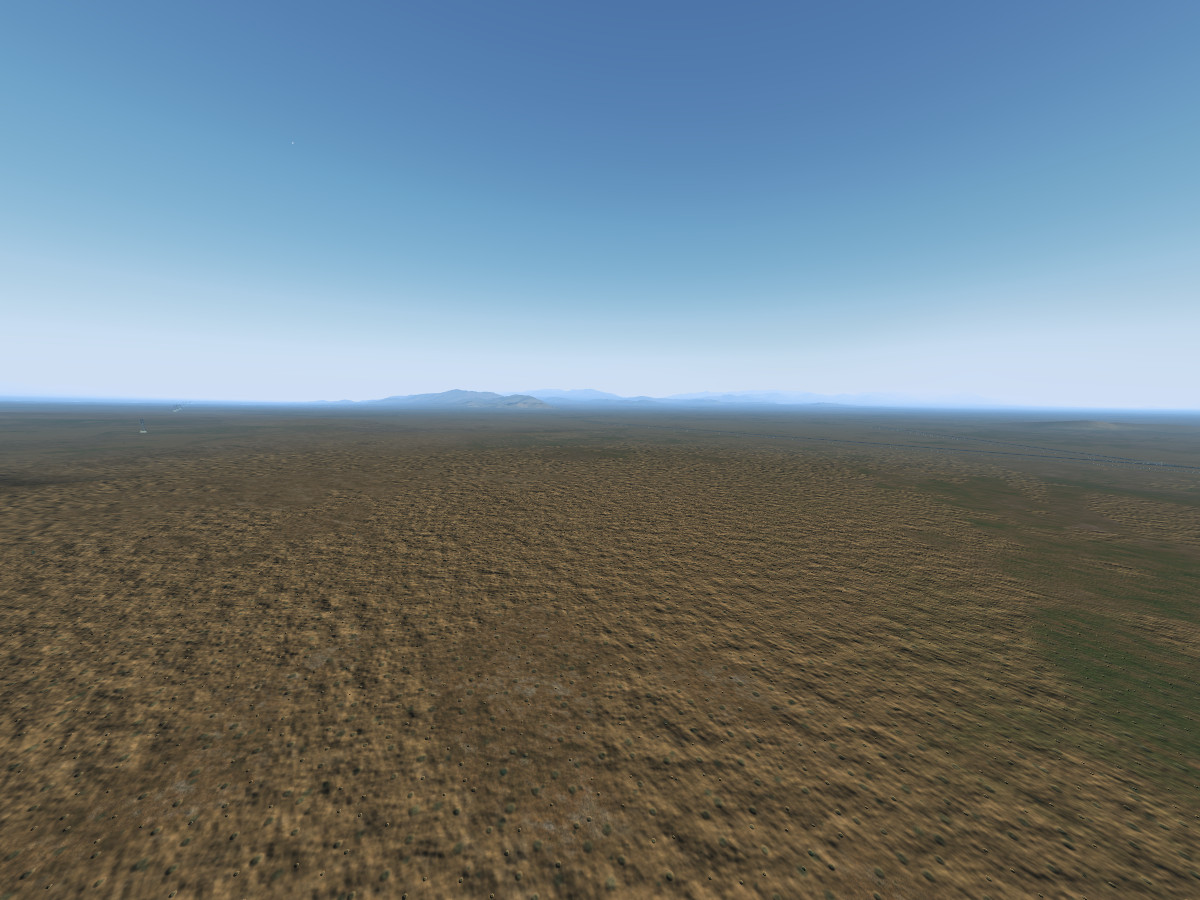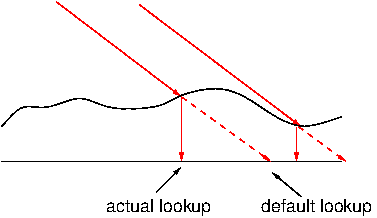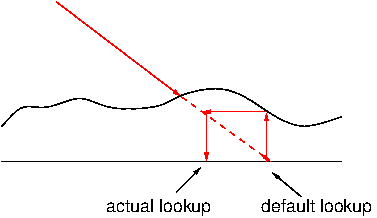# Relief maps

Often, we may want to render a surface that isn't precisely flat, but to model its actual surface structure in the mesh would be prohibitively expensive. Think for instance of a desert - there's small ripples of wind-blown sand, but if the sand isn't very fine, you can actually recognize each grain (or pebble) - however the idea to model every pebble by adding vertices is bound to fail soon.

The solution is, as in the case of other surface properties, to use a map to add apparent structure to a triangle between the mesh points via a texture (which can be a real texture or just procedural noise of course).

What we want to do is to create a surface that appears to have structure then. In order to accomplish that, we first need to understand the main visual cues for the perception of such structure.

• If the surface is lit with directional light, the way the light is strong when it falls directly onto a surface, attenuated when it reaches the surface with a shallow angle and shaded when the surface normal faces away from the light gives a strong visual indication of a relief.
• If a surface is textured with a fairly regular pattern, we see the original pattern when viewing the surface under a 90 degree angle but strong distortions if we look under a shallow angle. The way how the pattern (for instance individual pebbles) appears compressed and stretched to the eye due to the variation of view angle as we look at a relief provides a second depth cue (which is however absent for monochromatic surfaces).
• Finally, we can recognize a pronounced relief easily by the fact that parts of the relief more to the front can obscure the surface further back, i.e. block the line of sight.
Dependent on the desired accuracy and effect, different relief mapping techniques can be used. As can be seen by looking at the list of visual cues carefully, often they have to do with view angles, for which we need to know changes of the surface normal. Thus, unlike the scalar maps discussed in the previous section, relief maps are often vector maps. While the (rgba) channels of a texture are quite sufficient to store a 3-vector and then some, unlike for scalar maps, for vector maps we need to commit to a coordinate system in which the vector is supposed to be.

As the scalar maps, vector maps are the domain of the fragment shader,

## Bumpiness effect

Let's start with the simplest case - assume we have a terrain surface and we just want it to look a bit rough but are not picky about the detailed appearance. In principle, we'd need to know the normal as distorted by the bumps everywhere.

But, terrain being terrain, we know the normal n is usually pointing upward in suitable coordinates (say we have our model coordinates of the mesh arranged that way). The distortions are then going to be small wiggles around this upward direction. For small distortions and if we don't care about details, we can in fact change NdotL = dot(n, lightDir) rather than the normal itself. But we have to determine the magnitude of the distortion.

Say we have a noise function Noise2D(Pos.xy, scale) which takes a 2d position on the mesh and a length scale at which the noise is generated as arguments and returns a value between 0 and 1 as output. We can use that to model the displacement height of the terrain we want to render. Whether a surface is then lit or shaded depends of the steepness of that function along the light direction - mathematically the gradient of the heightfield.

This requires us to do a numerical derivative. We can do it as a finite difference, using fdot(x) = (f(x) - f(x+ Dx))/Dx. In numerical mathematics, that's usually a bad idea, but since we're not interested in the exact result anyway, this will do.

The algorithm then determines NdotL for a given position, computes the gradient of the heightfield by evaluating the noise function two times displaced along the light direction (all in model coordinates) and uses the result, multiplied with an overall noise_magnitude, to modify NdotL.

The relevant part of the fragment shader might then look like:

 NdotL = dot(n, lightDir); noisegrad = (Noise2D(Pos.xy, scale) - Noise2D(Pos.xy       + (0.05 * scale) * normalize(lightDir.xy),scale))/(0.05 * scale); NdotL = NdotL + noisegrad * noise_magnitude; if (NdotL > 0.0) {       color += diffuse_term * NdotL;       (...) }

(Note that the assumption that the terrain is close to flat has been used both in the fact that we characterize it by a 2d coordinate position and by the fact that we project the light direction into the (xy) plane via swizzling - the effect does not work too well for vertical rock faces.)

The result is a gentle pattern of light and shadow drawn on the terrain, giving the impression of a shallow relief.The simple bumpiness effect explained above using Perlin noise applied to terrain.

This is a technique which delivers a reasonable impression of roughness for very cheap, but offers little detailed control. If control over the apparent surface structure is needed (for instance when rendering a pattern of rivets on a wing), a normal map is the tool of choice.

## Normal mapping

For a normal map, we directly encode the surface normal in a texture rather than the displacement height over the terrain (in the example above, the distortion of the normal has been implicitly created by doing the derivative, but there's no mathematically clean relation between the heightfield and the actual distortion, it's just a 'look plausible').

We're going to pack the surface normal into the (rgb) channel of a texture now - but what coordinate system should that vector be in?

We can't encode a normal in eye space because this is not a fixed system, it rotates with the eye movement. Thus we could encode normals in model space such that directly vec3 normal = (texture2D(normalMapTex, gl_TexCoord.st)).rgb;. This works, but is a bit unwieldy. The normal map texture has to be different for every face of a cube even if the surface structure is supposed to be the same - because all the cubes are oriented differently. Moreover, every time we'd like to rotate or otherwise change a model in the 3d modeling application, the normal map texture has to be re-computed.

It's more usual to view a normal map as a property of the local surface (defined by the normal of the underlying triangle). Since that surface may be curved, we need a local coordinate system that follows the surface.

Such a coordinate system is provided by tangent space. The local surface is spanned by two vectors perpendicular to the local normal, the tangent and bitangent (sometimes also called binormal which are also perpendicular to each other such that all three vectors span a local orthonormal system. There are usually provisions to compute the set of tangents and binormals when a mesh is loaded on the C++ side of the application. Just as normals, tangents and binormals can then be attached to each vertex as attributes and fetched in the vertex shader. (Often it is also enough to attach just normal and tangent, because the binormal can then be obtained as the cross product of the two vectors, making use of the fact that they span an orthonormal system).

We can thus encode just the variation of the surface structure in the normal map, defining that the direction of the unperturbed normal is the z-coordinate and tangent and binormal stand for x and y. Note that the OpenGL side of the application needs to supply at least the tangent and in the example below also the binormal, it can't be obtained otherwise on the GLSL level.

The vertex shader then picks up the attributes, transforms them into eye space and declares tham as varying data types for interpolation across the triangle:

 attribute vec3 tangent; attribute vec3 binormal; varying vec3 eyeNormal; varying vec3 eyeTangent; varying vec3 eyeBinormal; (...) eyeNormal = normalize(gl_NormalMatrix * gl_Normal); eyeTangent = normalize(gl_NormalMatrix * tangent); eyeBinormal = normalize(gl_NormalMatrix * binormal); (...)

The fragment shader then picks up the interpolated values and uses the normal map to construct a normal by going along the direction of the coordinate axes given by the vector triplet:

 uniform sampler2D NormalTex; varying vec3 eyeNormal; varying vec3 eyeTangent; varying vec3 eyeBinormal; (...) vec4 normal_texel = texture2D(NormalTex, gl_TexCoord.st); N = normal_texel.rgb * 2.0 - 1.0; N = normalize(N.x * eyeTangent + N.y * eyeBinormal + N.z * eyeNormal); (...)

Note that since a texture can't encode negative color values but a normal can have a negative value, the texture (rgb) value encodes negative numbers in the range from 0-0.5 and positive values in the range from 0.5-1, and this is decoded in N = normal_texel.rgb * 2.0 - 1.0;. The normal N obtained at the end can now be used further down for lighting purposes.

## Parallax mapping

Parallax mapping is a cheap way to implement the effect that a regular texture structure appears compressed and stretched dependent on view angle when there is surface bumpiness. It is in essence a prescription to look up a texture at a position different from the nominal reference point.

For this purpose, the bumpiness is characterized by a heightfield over the nominal triangle (in the following, we discuss it for a surface that is stretched in the (xy) plane in model space for simplicity, but it can be generalized to tangent space easily using the techniques described above).

The idea is as follows: We'd like to look up the texture where the view ray intersects the heightfield (and you can see how the stretching arises from this procedure):Parallax mapping - ideal.

The problem is, we don't readily know where this happens. So instead, we use the heightfield at the default lookup position to estimate how the heightfield looks like, go back by and offset to the original view ray and look up at that position. This doesn't give the exact result, but if the heightfield is not strongly varying, it is close enough to get compelling visuals.Parallax mapping - real.

To aviod artifacts at shallow viewing angles, we may want to limit the offset at which we retrieve the texture to some fixed value.

If the view vector in model coordinates is view_vec and we have a heightfield function hfield(in vec2 xy) (which may be noise or a texture) that returns the displacement over the default surface, the texture lookup offset is

 vec2 texCoord = gl_TexCoord.st; texCoord = texCoord + hfield(texCoord) * view_vec.xy;

And surprisingly that's already all that's needed - look up a texture at the shifted coordinates and it will show a pattern distortion consistent with the heightfield.

## Height mapping

If we want to do better, we need to determine the actual intersection between view ray and heightfield. There's many different techniques to do that (all involve repeated calls to the heightfield function) - one can do adaptive subdivision and zero in for instance, or just do a straightforward outward-in sampling. What is the best technique depends on what accuracy is needed and most important how the heightfield looks like. A sharp heightfield in which strongly defined cube-like clusters reach upward requires different techniques than a gently rolling hillscape.

The following algorithm requires us to have a heightmap texture in which we want to map to a size given by relief_hresolution and for which we want that the vertical size is mapped to relief_vscale.

The fact that the relief can only be a certain scale above the ground is used in the following algorithm to find a starting point for a search along the view ray (given by the coordinate difference relPos). The heightfield is evaluated at trial positions progressively inward till an intersection is found, that value is then returned as the shift in the texture coordinate.

Functions implementing this in the fragment shader might look like:

 uniform float relief_hresolution; uniform float relief_vscale; uniform sampler2D heightmap_texture; const int npoints = 50; float height_function (in vec2 pos) { vec4 height_texel = texture2D (heightmap_texture, pos/relief_hresolution); return relief_vscale * height_texel.a; } vec2 height_coord_shift (in vec2 base_pos) { vec3 nRay = normalize(relPos); float sampling_resolution = relief_vscale/float(npoints) * 0.6; vec3 vRay = -sampling_resolution * nRay; vec3 pos = vec3(base_pos, 0.0); float height = 0.0; int flag = 0; pos += vRay * float(npoints); for (int i = 0; i < npoints; i++)     {     height = height_function (pos.xy);     if (height > pos.z)        {        break;        }     pos -= vRay;     } return pos.xy - base_pos.xy; } (...) vec2 texCoord = gl_TexCoord.st; texCoord = texCoord + height_coord_shift(texCoord);

Since only the alpha channel of the texture is needed to provide the heightfield, the (rgb) channels can carry the associated normal map for lighting purposes (it can also be computed from the heightfield inside the shader, but that's more expensive than to use the existing texture lookup). The results of a heightmap are quie compelling if the view angle is not too shallow - the texture pattern shows the right distortions and compelling shadows and bumps can obscure what is behind.A heightmap applied to the terrain mesh.

In fact all visual cues are there - however note that the heightmap does just that - it does not alter the mesh, so from the point of e.g. collision detection the terrain is unchanged - one can't 'walk' on a heightmap, only on the original mesh.

Also, texture lookup calls are modestly expensive - and the need for perhaps 10-20 calls to sample the intersection point with good accuracy is something that makes a heightmap a performance-costly technique.

Continue with Light intensity.

Back to main index     Back to rendering     Back to GLSL

Created by Thorsten Renk 2016 - see the disclaimer, privacy statement and contact information.